### Question 9. Solve the following example. An object takes 5 s to reach the ground from a height of 5 m on a planet. What is the value of g on the planet?

Question 9.

Solve the following example.

An object takes 5 s to reach the ground from a height of 5 m on a planet. What is the value of g on the planet?

Answer:

Given;

Time for object to reach ground (t) = 5 s;

Height of object from the ground(s) = 5m;

Acceleration due to gravity (g) =? ;

Since when Object is released from the height of 5m it will be in free fall hence its initial velocity (u) = 0;

Now, By Newton’s second equation of motion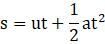Here u = 0, a = g, t = 5s, s = 5m;

Putting these values in the above equation we get;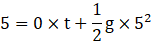⇒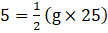.

⇒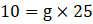.

⇒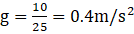.

Hence acceleration due to gravity on the planet is 0.4m/s2.